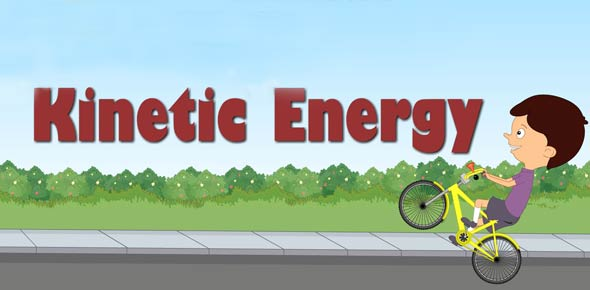# Lesson 1 : Basic Terminology And Concepts

20 Questions | Total Attempts: 44SettingsThis tackles about Work, Potential Energy, Kinetic Energy, Mechanical Energy and Power.

• 1.
There are three key ingredients to work - force, displacement, and __________.
• 2.
__________ is the stored energy of position possessed by an object.
• 3.
__________ is the energy stored in an object as the result of its vertical position or height. The energy is stored as the result of the gravitational attraction of the Earth for the object.
• 4.
__________ is the energy stored in elastic materials as the result of their stretching or compressing.
• 5.
An object that has motion - whether it is vertical or horizontal motion - has __________ .
• 6.
__________ is the energy that is possessed by an object due to its motion or due to its position.
• 7.
Mechanical energy can be either kinetic energy ( __________ ) or potential energy (stored energy of position).
• 8.
An object that possesses mechanical energy is able to do __________.
• 9.
The standard metric unit of power is the __________.
• 10.
The expression for power is __________.
• 11.
There are two forms of potential energy discussed in our course - gravitational potential energy and __________.
• 12.
The standard metric unit of Energy is the __________.
• 13.
In terms of potential energy, the equilibrium position could be called the __________.
• 14.
The total amount of mechanical energy is merely the sum of the __________ and the kinetic energy.
• 15.
An object possesses gravitational potential energy if it is positioned at a height above (or below) the __________ height.
• 16.
Two physics students, Will N. Andable and Ben Pumpiniron, are in the weightlifting room. Will lifts the 100-pound barbell over his head 10 times in one minute; Ben lifts the 100-pound barbell over his head 10 times in 10 seconds.  Which student delivers the most power? ______________
• 17.
Two physics students, Will N. Andable and Ben Pumpiniron, are in the weightlifting room. Will lifts the 100-pound barbell over his head 10 times in one minute; Ben lifts the 100-pound barbell over his head 10 times in 10 seconds. Which student does the most work? ______________
• 18.
A tired squirrel (mass of approximately 1 kg) does push-ups by applying a force to elevate its center-of-mass by 5 cm in order to do a mere 0.50 Joule of work. If the tired squirrel does all this work in 2 seconds, then determine its power.
• A.

0.25 Watts

• B.

0.75 Watts

• C.

1.00 Watts

• D.

None of the above

• 19.
When doing a chin-up, a physics student lifts her 42.0-kg body a distance of 0.25 meters in 2 seconds. What is the power delivered by the student's biceps?
• A.

51.5 Watts

• B.

75.0 Watts

• C.

25.0 Watts

• D.

None of the above.

• 20.
An escalator is used to move 20 passengers every minute from the first floor of a department store to the second. The second floor is located 5.20 meters above the first floor. The average passenger's mass is 54.9 kg. Determine the power requirement of the escalator in order to move this number of passengers in this amount of time.
• A.

1000 W

• B.

933 W

• C.

879 W

• D.

None of the above.

Related TopicsBack to top